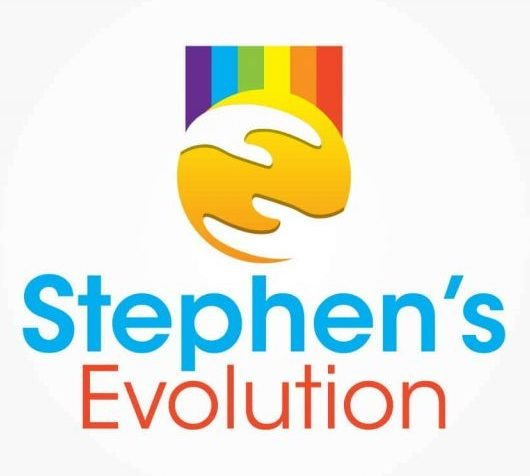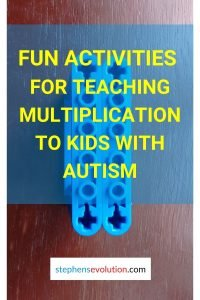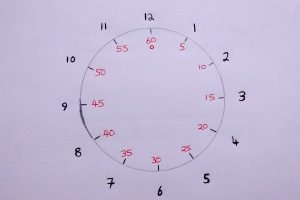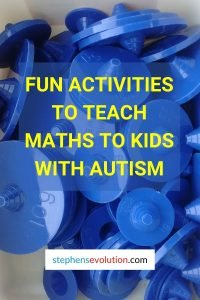# Ways you can teach maths to children with autism

Stephen McHugh
This post was last updated on
August 19, 2022
Category :

Mathematics can be a difficult enough subject at times for us all, with many different concepts to learn and apply to problems of everyday life. Given my language development delays as a result of being on the autism spectrum, it meant there would be times when I’d have difficulties understanding new concepts. Consequently varying degrees of support were necessary to help me to consolidate topics, apply new knowledge and move from one area of the curriculum to another. The steps outlined in this post are what benefited me the most. They may be of benefit to others, they may not, since everybody is different and unique. If this post inspires ideas of your own, that would be great too.

I became comfortable in carrying out addition and subtraction throughout infant school. Despite me having a liking for numbers, my sum writing was rather patchy. One way I felt comfortable doing sums like 4 + 5 would be to have 4 objects, add a further 5 and count 9 of them altogether. For 8 - 6 I’d take out 8 objects, take away 6 to leave 2. Up until about 5 years of age, I was only able to count up to 59, perhaps from clocks and telling the time, before my mother introduced me to numbers beyond.

Once I was comfortable working with sums involving numbers up to 100 using money-related matters, I was given the opportunity to pay for items whenever we went out shopping. Whenever there were lots of spare coins around the house, I liked to count them to see how much they added up to. At the time, I felt more comfortable trying to do calculations mentally rather than writing down working out on paper. The following methods below proved easier for me to grasp addition and subtraction, especially as it can be possible to visualise the processes. You're welcome to check out a link here I found on Youtube, where these concepts are explained clearly.  Objects are shown to be added and taken away from fixed numbers of them.

You can also use what's called an abacus for addition and subtraction, an old counting frame with 10 rows consisting of 10 beads each.

45 + 33 : 40 + 30 + 5 + 3 = 78 ( 4 tens + 5 units + 3 tens + 3 units)

Draw 4 blocks each representing 10 along with 5 smaller ones representing 1 each.

Draw 3 more blocks each representing 10 along with 3 smaller ones representing 1 each.

You should end up with 7 blocks representing 10 each and 8 smaller ones indicating 1 each, giving 78.

If the number of units ever equals 10 or more, you should add an extra 1 to the total number of tens, and take away 10 from the total number of units.

### Subtraction

45 - 33 : (40 - 30) + (5 - 3 = 12) ( 4 tens + 5 units - 3 tens - 3 units)

Draw 4 blocks each representing 10 along with 5 smaller ones representing 1 each.

Cross out 3 blocks representing 10 along with 3 smaller ones indicating 1 each.

You should be left with 1 block representing 10 and 2 units, leaving 12.

If the number of units is -1 or less,  you should add 10 to the total number of units, and take away an extra 1 from the total number of tens.

## Multiplication and division

### MultiplicationPin

During the early part of my life in junior school, seeing multiplication signs for the first time left me confused as to what they meant. One strategy I employed for learning my tables was to read them over and over and test myself. This paid dividends when it came to class spelling and tables tests because it helped to put the boys into the lead over the girls. Using the two LEGO pieces side by side in the picture to the right, there are 2 studs across and 4 down. Therefore, for 4 multiplied by 2 we get 8. We can see from the picture it is 4 lots of 2(2+2+2+2), or 2 lots of 4(4+4). Further ideas for using LEGO to teach maths can be found here.

About 1 year later, and still needing a recap about how multiplication worked we had to individually recite the tables as far as we could. The furthest we were allowed to go was up to 12. After listening to others before me in the class register patterns became more and more discernible to me in time for my turn. This approach proved successful, as I was able to say my times tables from 2 to 12. My preoccupation with counting sometimes led to me spending periods of time pressing the equals sign on the calculator numerous times. This gave me an insight into number patterns, e.g.  10, 20, 30, 40 etc.

Judging by my year 5 (junior 3) teacher’s teaching styles, I believe they’d have used methodologies similar to the ones to teach me multiplication and division shown, and addition and subtraction above.I had a great interest in clocks. These can be used to an extent to give one an insight into the times tables, particularly the 5 times table. As shown in the drawing to the left, one could label the seconds section by placing 5 at 1, 10 at 2, 15 at 3, until 60 at 12.

### Division

Like multiplication, division was somewhat confusing for me at first, especially ones involving remainders. An example can be sharing out 25 sweets amongst 5 children(25 divided by 5). Have 25 small objects, e.g. marbles to represent sweets and small toy models to represent children. The idea here is to place 1 marble at each model until everyone of them has 1. Repeat the process until there are no more marbles to share out equally amongst the 5 models. Here, there should be enough to give each model 5 marbles each.

Another way I got started in division was using what’s known as the chunk method, useful for larger division sums. Take 15 ÷  5 for example. Here I’d have 15 objects and take away 5 from 15 to leave 10, take away 5 again from 10 to leave 5 remaining; and for a third time take away the remaining 5 to leave 0. Therefore the answer to 15 ÷ 5 = 3, since we take away 5 objects 3 times from 15 at a time. By using an abacus here, you could count a separate group of 15 beads, and then move groups of 5 away at a time to leave 0. It should take you 3 repetitions to do this.

If, on the other hand, there were 24 sweets to be shared out amongst 5 of you, only 4 can have five each with 4 remaining. Think about applying a bit of drama here, which is probably what my junior 3 (year 5) teacher would have encouraged me to do. For example, if there are 4 children with 5 sweets at the end of the sharing and you were the unfortunate child with only 4 sweets, you’ll naturally be unhappy, saying, “That’s not fair, all the rest have 5 and I only have 4.”

### Long multiplication

25 x 35   (25 x 30) + (25 x 5) = 750 + 125 = 875

25 x 5 = 125; 25 x 30 = 750 (25 x 3 x 10)

Above is the method which I learned in junior 3(year 5) which helped me to develop good mental arithmetic skills over time, especially when it all became second nature to me.

## Ratios

Ratios appeared a little confusing to me at first, until my dad pointed out that fractions and ratios are quite similar with no remainders involved.

100 : 25 can be simplified to 4 : 1 since 25 x 4 = 100

10 : 2 can be simplified to 5 : 1 since 2 x 5 = 10

5 : 3 cannot be simplified because 5 doesn’t have 3 as a factor (5 cannot be divided by 3 without remainders). We can see that from 3 x 1 = 3 and 3 x 2 = 6. Number 5 can be divided by is 1 and itself only, making it a prime number.

## Decimals

With decimals, what set me on my way here was that you moved the decimal point to the right in multiplication and to the left during division.  Eventually I recognised the number of times you move the decimal point is related to the number of zeros as shown below.

Multiplying by 10 involves moving the decimal point 1 place to the right.

e.g. 0.12345 x 10 = 1.2345  and  0.00123 x 10 = 0.0123

Multiplying by 100 involves moving the decimal point 2 places to the right

e.g. 0.12345 x 100 = 12.345 and 0.00123 x 100 = 0.123

Note how the number of times you move the decimal point to the right equates to the number of zeros you remove after the decimal point.

Dividing by 10 involves moving the decimal point 1 place to the left

e.g. 123.45/10 = 12.345; and 0.006789/10 = 0.06789

Dividing by 100 involves moving the decimal point 2 places to the left

e.g. 123.45/100 = 1.2345; and 0.006789/100 = 0.6789

Note how the number of times you move the decimal point to the left equates to the number of zeros you remove after the decimal point.

## Fractions

Looking at the fractions below, these probably would have been the approaches of my junior 3 (year 5) teacher. Here it was easy to see what fractions look like compared to each other from a visual point of view.

 1, 1/1, 2/2, 3/3 or 4/4

 1/2 1/2

1/2 + 1/2 = 2/2 = 1

 1/3 1/3 1/3

1/3 + 1/3 = 2/3

1/3 + 1/3 + 1/3 = 3/3 = 1

 1/4 1/4 1/4 1/4

1/4 + 1/4 = 2/4 = 1/2

1/4 + 1/4 + 1/4 = 3/4

1/4 + 1/4 + 1/4 + 1/4 =  4/4 = 1

In both instances below, a and b are used in place of numbers. They could represent any number at all.

• To find 1/b of something divide that something by b.
• To find a/b of something divide that something by b first and multiply by a.

### Fraction simplification

Astronomy was, and still is, a strong interest of mine. I once had a telescope which had a choice of magnifications of 15x, 30x, 45x and 60x. Here, the list of fractions can be 15/30, 15/45, 15/60, 30/45, 30/60 and 45/60. As we can see below, fractions such as these can be simplified if we can work out what number to divide the top and bottom numbers by.

15/30 = 1/2   Both can be divided by 15 (15/15 =1 and 30/15 =2)

15/45 = 1/3   Both can be divided by 15 (15/15 =1 and 45/15 =3)

15/60 = 1/4   Both can be divided by 15 (15/15 =1 and 60/15 =4)

30/45 = 2/3  Both can be divided by 15 (30/15 =2 and 45/15 =3)

30/60 = 1/2  Both can be divided by 30 (30/30 =1 and 60/30 =2)

45/60 = 3/4  Both can be divided by 15 (45/15 =3 and 60/15 =4)

## Fun activities to aid learning and understanding

Measurements attracted my attention very quickly. Whenever there was a balance within reach, I liked to try and get them to balance by placing equal weights on either side. I often wanted to know how much the concrete of our driveway weighed. Guided by my mother, it was all about finding the volume of concrete used, along with its density(grams per cm cubed). The value I think was near to 3,500 kg, though I don’t know how accurate that actually was.

Outdoors, in the back garden I kicked and hit objects up into the air (balls especially), and estimated how high they’d go. Once I got estimates I enjoyed the challenge of converting feet to metres. A jug that had over 1,000 millilitres(1 litre) printed on a scale on the jug’s side gave me an insight into the comparison of how many millilitres equalled how many pints. I admit to having a great  interest in measurements of things, particularly heights of tall people and objects, and somethings their weights. Whenever I found a balance, I liked to try and get them to balance by placing equal weights on either side.If you have access to a balance and any weights, why not try this. If you placed 20 grams on one side using 2 x 10 gram weights, you should be able to balance it by putting 4 x 5 gram weights on the opposite side.I longed to work out a relationship between miles and kilometres. According to relevant data, 3 miles is approximately 5 km and did 5 + 3 to get 8 km for 5 miles. After carrying out a second calculation of 5 + 8 = 13 to get 13 km for 8 miles a pattern was slowly emerging for me. Here are slightly more accurate figures, 3 miles = 4.8 km, 5 miles = 8 km and 8 miles = 12.8 km. So my solutions here were, coincidentally, quite precise.

During my junior school years we did mathematical problems relating to snooker scores. At home we had relatively small sized snooker and pool tables at home. Here, I felt inspired to pot balls and add up the value of each ball potted to help improve my mental arithmetic, sometimes using my own values for certain balls.

I had an interest in counters, especially ones on measuring devices such as speedometers and mileometers. Other counters included video recorders showing how many seconds a particular film or recording has been playing. At school there were trundle wheels with counters on them that displayed the values of lengths and widths of things. I don't think I ever got to use one of them, but if I could have, one task would have been to measure the length of a running track on the school field. The objective here would be to calculate speeds of those doing running races.

As you may have read earlier, space was another interest I developed, evidenced by a height measuring chart at home displaying the solar system. Seeing all the facts about the planets, I divided their respective distances from the sun by their heights on the scale to work out my own distance scale.

## It's alright to make mistakes

I do admit to being too focused on perfection too, partly because of the fear of being told off for doing something wrong. Seeing my older brothers’ mistakes helped me to have more confidence in myself and not to feel too disheartened about making mistakes.' According to him it was all about getting the right answers. He went along with the ‘game’, whereas I didn’t. It was a case of me trying to understand how things worked in general. Whenever I felt I'd mastered something difficult, I liked to keep going on at it, whilst rejoicing at overcoming what I'd considered to be great barriers, especially if I received praise and encouragement.  Read this blog post here from another person on the autism spectrum regarding their views about imperfectionism. And furthermore, making errors can be good for science too. See this link here to a section on the bbc website.

## Conclusion

For those with autism and language development delays, understanding new mathematical concepts can prove to be very challenging. However, with the right approaches, which can include targeting one's interests and even using visual aids, adequate progress can be made, and the foundations laid for the next learning step. And finally, making mistakes is alright, but remember to view them as learning opportunities.  They can be important for future successes. Read about this in this blog post here.